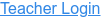### Topics

• Graphing Linear Equations
• Lines
• Slope

### Description

Explore the world of lines. Investigate the relationships between linear equations, slope, and graphs of lines. Challenge yourself in the line game!

### Sample Learning Goals

• Explain how the slope of a graphed line can be computed.
• Graph a line given an equation in either slope-intercept or point-slope form.
• Write an equation in slope-intercept or point-slope form given a graphed line.
• Predict how changing variables in a linear equation will affect the graphed line.
Version 1.2.2